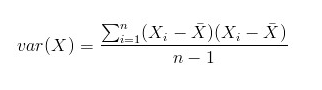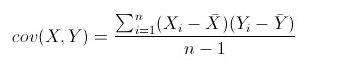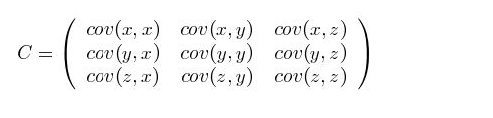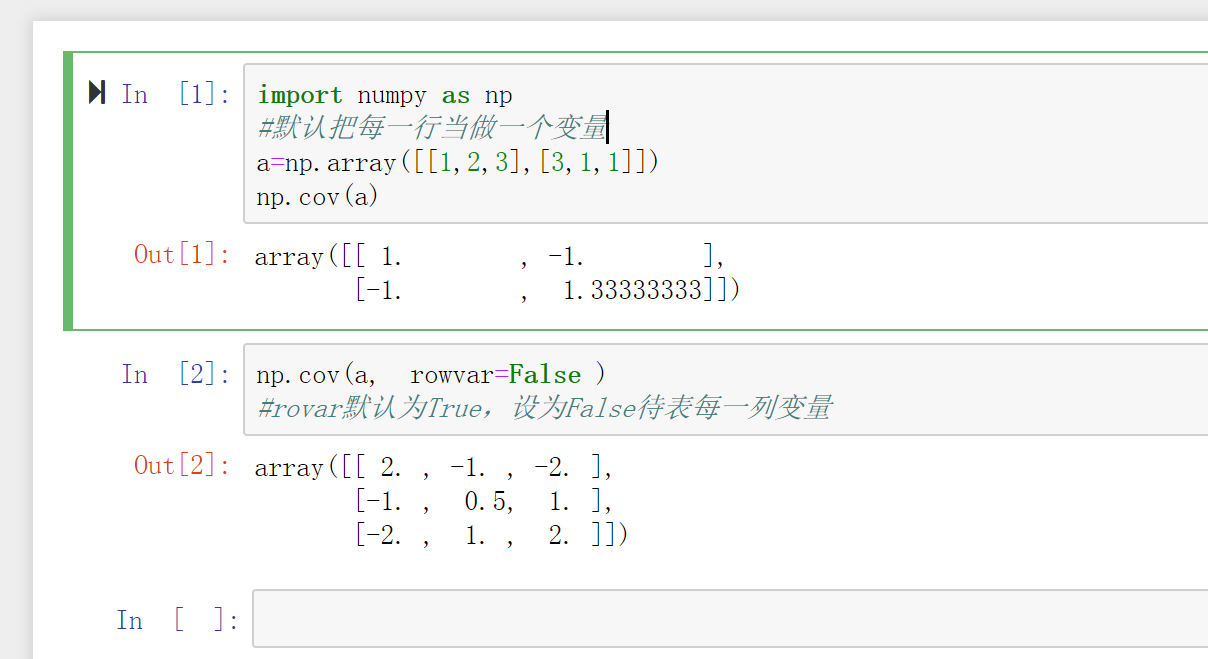• numpy协方差矩阵numpy.cov numpy.cov(m, y=None, rowvar=True, bias=False, ddof=None, fweights=None, aweights=None)[source] Estimate a covariance matrix, given data and weights. ...

numpy协方差矩阵numpy.cov

numpy.cov(m, y=None, rowvar=True, bias=False, ddof=None, fweights=None, aweights=None)[source]

Estimate a covariance matrix, given data and weights.
Covariance indicates the level to which two variables vary together. If we examine N-dimensional samples,, then the covariance matrix elementis the covariance ofand. The elementis the variance of.
See the notes for an outline of the algorithm.
Parameters: m : array_like

A 1-D or 2-D array containing multiple variables and observations. Each row （行） of m represents a variable（变量）, and each column（列） a single observation of all those variables（样本）. Also see rowvar below.
y : array_like, optional

An additional set of variables and observations. y has the same form as that of m.
rowvar : bool, optional

If rowvar is True (default), then each row represents a variable, with observations in the columns. Otherwise, the relationship is transposed: each column represents a variable, while the rows contain observations.
bias : bool, optional

Default normalization (False) is by (N - 1), where N is the number of observations given (unbiased estimate). If bias is True, then normalization is by N. These values can be overridden by using the keyword ddof in numpy versions >= 1.5.
ddof : int, optional

If not None the default value implied by bias is overridden. Note that ddof=1 will return the unbiased estimate, even if both fweights and aweights are specified, and ddof=0 will return the simple average. See the notes for the details. The default value is None.

New in version 1.5.

fweights : array_like, int, optional

1-D array of integer freguency weights; the number of times each observation vector should be repeated.

New in version 1.10.

aweights : array_like, optional

1-D array of observation vector weights. These relative weights are typically large for observations considered “important” and smaller for observations considered less “important”. If ddof=0 the array of weights can be used to assign probabilities to observation vectors.

New in version 1.10.

Returns: out : ndarray

The covariance matrix of the variables.

corrcoef

Normalized covariance matrix

Notes
Assume that the observations are in the columns of the observation array m and let f = fweights and a = aweights for brevity. The steps to compute the weighted covariance are as follows:

>>> w = f * a >>> v1 = np.sum(w) >>> v2 = np.sum(w * a) >>> m -= np.sum(m * w, axis=1, keepdims=True) / v1 >>> cov = np.dot(m * w, m.T) * v1 / (v1**2 - ddof * v2)

Note that when a == 1, the normalization factor v1 / (v1**2 - ddof * v2) goes over to 1 / (np.sum(f) - ddof) as it should.
Examples
Consider two variables,and, which correlate perfectly, but in opposite directions:

>>> x = np.array([[0, 2], [1, 1], [2, 0]]).T >>> x array([[0, 1, 2],  [2, 1, 0]])

Note howincreases whiledecreases. The covariance matrix shows this clearly:

>>> np.cov(x) array([[ 1., -1.],  [-1., 1.]])

Note that element, which shows the correlation betweenand, is negative.
Further, note how x and y are combined:

>>> x = [-2.1, -1, 4.3] >>> y = [3, 1.1, 0.12] >>> X = np.stack((x, y), axis=0) >>> print(np.cov(X)) [[ 11.71 -4.286 ]  [ -4.286 2.14413333]] >>> print(np.cov(x, y)) [[ 11.71 -4.286 ]  [ -4.286 2.14413333]] >>> print(np.cov(x)) 11.71
总结
理解协方差矩阵的关键就在于牢记它的计算是不同维度之间的协方差，而不是不同样本之间。拿到一个样本矩阵，最先要明确的就是一行是一个样本还是一个维度，心中明确整个计算过程就会顺流而下，这么一来就不会迷茫了。

posted on 2018-04-01 22:20 多一点 阅读(...) 评论(...) 编辑 收藏

展开全文• I am using numpy and want to compute the covariance matrix for an ... I am trying to use numpy.cov() but am not getting the correct results. More details below.My ndarray is 768x8 for where 8 is ...I am using numpy and want to compute the covariance matrix for an ndarray. I am trying to use numpy.cov() but am not getting the correct results. More details below.
My ndarray is 768x8 for where 8 is the numbers features in my data set.
When I use MATLAB to compute the covariance matrix, I get a 8x8 (which is what I require), but when I use np.cov(), I get a 768x768 which is incorrect. I tried changing the rowvar argument to true and this does not work.
What would be the correct call to numpy.cov()? In other words, how would I reproduce the cov() results from MATLAB using numpy.
解决方案
Amazingly, the documentation might tell you. You should pass rowvar=False to indicate that columns represent variables.
>>> data.shape
(768, 8)
>>> numpy.cov(data, rowvar=False).shape
(8, 8)

展开全文• Numpy中的 cov() 可以直接求得矩阵的协方差矩阵。 先简单概述一下什么是协方差： 在概率论和统计学中，协方差用于衡量两个变量的总体误差。而方差是协方差的一种特殊情况，即当两个变量是相同的情况。 期望值分别为E...
1. 概述
Numpy中的 cov() 可以直接求得矩阵的协方差矩阵。 先简单概述一下什么是协方差： 在概率论和统计学中，协方差用于衡量两个变量的总体误差。而方差是协方差的一种特殊情况，即当两个变量是相同的情况。 期望值分别为E[X]与E[Y]的两个实随机变量X与Y之间的协方差Cov(X,Y)定义为：从直观上来看，协方差表示的是两个变量总体误差的期望。
2. numpy.cov()
2.1 语法
numpy.cov(m, y=None, rowvar=True) 给定数据和权重，估计协方差矩阵。
协方差表示两个变量一起变化的水平。 如果我们检查N维样本，X = [x_1，x_2，… x_N] ^ T，则协方差矩阵元素C_ {ij}是x_i和x_j的协方差。 元素C_ {ii}是x_i的方差。
2.2 参数：
m：array_like 包含多个变量和观测值的1-D或2-D数组。 m的每一行代表一个变量，每一列都是对所有这些变量的单一观察。
y：array_like，可选 另外一组变量和观察。 y具有与m相同的形式。
rowvar：布尔值，可选 如果rowvar为True（默认值），则每行代表一个变量X，另一个行为变量Y。否则，转换关系：每列代表一个变量X，另一个列为变量Y。
随着版本的变化，函数的参数也在更新，更多详情点击 查看。 返回： 变量的协方差矩阵
2.3 实例
#!/usr/bin/env python3
# -*- coding: utf-8 -*-
# @Time    : 2019/3/1 17:06
# @Author  : Arrow and Bullet
# @FileName: cov().py
# @Software: PyCharm
# @Blog    ：https://blog.csdn.net/qq_41800366
from numpy import *

A = array([[0, 2], [1, 1], [2, 0]]).T
print(A)  # [[0 1 2][2 1 0]]
B = cov(A)  # 默认行为变量计算方式，即X为行，Y也为行
print(B)  # [[ 1. -1.][-1.  1.]]
C = cov(A, rowvar=False)  # 此时列为变量计算方式 即X为列，Y也为列
print(C)  # [[ 2.  0. -2.][ 0.  0.  0.][-2.  0.  2.]]

希望能够帮助到大家，有什么问题可以 直接评论即可，喜欢有用的话可以点个赞让更多的人看到，如果不够详细的话也可以说，我会及时回复的。
展开全文协方差 python中协方差
• 1、关于方差与协方差 方差公式： 方差度量 协方差公式： 协方差矩阵的介绍和计算见： https://blog.csdn.net/Mr_HHH/article/details/78490576 2、numpy.cov()函数
1、关于方差与协方差
方差公式： 方差度量协方差公式：协方差矩阵的介绍和计算见： https://blog.csdn.net/Mr_HHH/article/details/78490576
数据集若有，三个位度（三个特征）协方差应该如下：2、numpy.cov()函数3、意义
协方差的为正，代表两个变量正相关；负，代表两个变量负相关


展开全文协方差
• 协方差：numpy.cov官网参数 从数值来看，协方差的数值越大，两个变量同向程度也就越大。反之亦然。 numpy.cov(m, y=None, rowvar=True, bias=False, ddof=None, fweights=None, aweights=None)[source]...
• ## numpy中cov()的计算

千次阅读 2019-05-31 14:40:35
协方差公式： 上面的公式计算协方差的一般公式 对于样本矩阵，有N个分量, 则 ...import numpy as np x = np.array([[0, 2], [1, 1], [2, 0]]).T x array([[0, 1, 2], [2, 1, 0]]) np.cov(x) array([[ 1., -...协方差矩阵
• ## numpy.cov()和numpy.var()的用法

万次阅读 多人点赞 2017-10-31 09:21:28
在PCA中涉及到了方差var和协方差cov，这里简单总结下。 首先：均值，样本方差，样本协方差的公式为均值：X¯=1N∑Ni=1Xi\bar{X}=\frac{1}{N} \sum_{i=1}^{N}X_i样本方差：S=1N−1∑Ni=1(Xi−X¯)2S=\frac{1}{N-1} \...var matlab pca
• numpy.cov(m, y=None, rowvar=True, bias=False, ddof=None, fweights=None, aweights=None) 对给定的数据和权重，估计协方差矩阵 协方差表示两个变量在一起变化的水平。如果我们检查N维样本，则X = [x_1，x...python 协方差矩阵
• These values can be overridden by using the keyword ddof in numpy versions >= 1.5. ddof : int, optional If not None the default value implied by bias is overridden. Note that ddof=1 will return the ...
• ## numpy.cov以及corrcoef

千次阅读 2017-02-15 11:01:55
Cov（X，Y）=E[（X-E（X））（Y-E（Y））] #Estimate a covariance matrix, given data and weights. 给定数据和权重，求解协方差矩阵# Covariance indicates the level to which two variables vary together. If ...
• 在计算协方差矩阵时,我使用numpy.covnumpy.dot得出了不同的结果.如果我的二维数组是(5,9),我想得到一个5×5(即NxN)协方差矩阵.这是我使用numpy.dot获得的.使用numpy.cov,我得到的协方差矩阵为9×9.这与我所需的...
• numpy.cov()的作用是计算协方差矩阵，下面给出几个例子 >>> x = np.array([[0, 2], [1, 1], [2, 0]]).T >>> x array([[0, 1, 2], [2, 1, 0]]) >>> np.cov(x) array([[ 1., -1.], [-1., 1.]]) >>> x =python
• 1.命令numpy.cov(m, y=None, rowvar=True, bias=False, ddof=None, fweights=None, aweights=None)2.参数Parameters ---------- m : 一维或2维数组，每行代表一个特征，每列代表一个样本  y : 数组，可选 额外...
• 在PCA中会用到，记录一下numpy.cov()的作用是计算协方差矩阵，下面给出几个例子>>> x = np.array([[0, 2], [1, 1], [2, 0]]).T>>> xarray([[0, 1, 2],[2, 1, 0]])>>> np.cov(x)array([[ 1., -1.],[-1., 1.]])>>> x =...
• 协方差提供了两个变量或更多组...如果COV(xi，xj)= 0，则变量不相关如果COV(xi，xj)> 0，则变量呈正相关如果COV(xi，xj)>用法：numpy.cov(m, y=None, rowvar=True, bias=False, ddof=None, fweights=None, aweight...
• 这里使用numpy库的cov函数来计算协方差，输出结果为一个协方差矩阵，results[i][j]表示第i个变量与第j个变量的协方差,比如np.cov(x)的结果为 >>> np.cov(x) array( [[ 1. , 0. ], [ 0. , 0.33333333]] ) x...corrcoef python 协方差 相关系数...